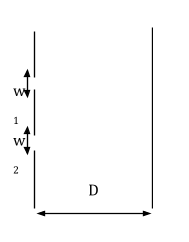# In Young’s double-slit experiment, the width of one of the slits is three times the other slit. The amplitude of the light coming from a slit is proportional to the slit-width. Find the ratio of the maximum to the minimum intensity in the interference pattern.

a. 4: 1

b. 2: 1

c. 3: 1

d. 1: 4Given: w2 = 3w1

Also, A ∝ w

w1 / w2 = ⅓ … (1)

Assume w1 = x, w2 = 3x

We know that

Imax = (A1 + A2)2, and

Imin = (A1 – A2)2

A1 / A2 = w1 / w2 ….(2)

From equation (2) we can say that

A1 = A and A2 = 3A

Now, $$\frac{I_{max}}{I_{min}} = \frac{(A+3A)^{2}}{(A – 3A)^{2}} = \frac{16A^{2}}{4A^{2}} = \frac{4}{1}$$

⇒ $$\frac{I_{max}}{I_{min}} = \frac{4}{1}$$(0)(0)